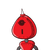# construct a parallelogram whose diagonals are 5.4cm and 6.2 cm and an angle between them is 70°

construct a parallelogram whose diagonals are 5.4cm and 6.2 cm and an angle between them is 70°

### 1 thought on “construct a parallelogram whose diagonals are 5.4cm and 6.2 cm and an angle between them is 70°”

1.Step-by-step explanation:

Steps of Construction of a parallelogram:

(i) Draw AC = 5.4 cm.

(ii) Bisect AC at O.

(iii) Make ∠COX = 70° and produce XO to Y.

(iv) Set off OB = 1/2 (6.2) = 3.1 cm and OD = 1/2 (6.2) =3.1cm .

(v) Join AB, BC, CD and DA. Then, ABCD is the required parallelogram